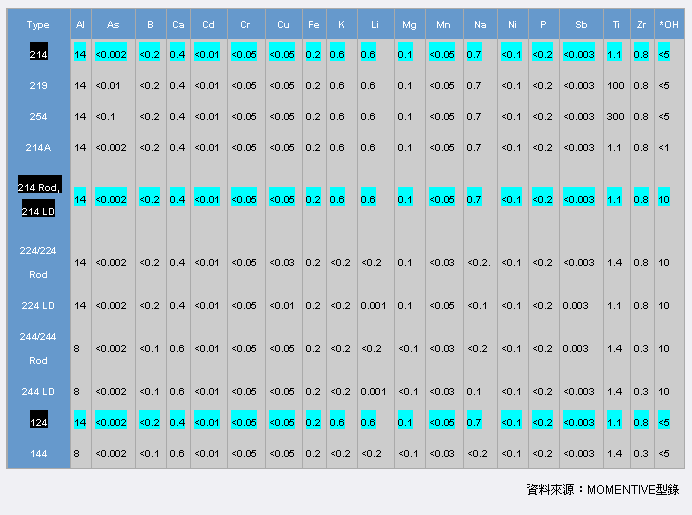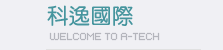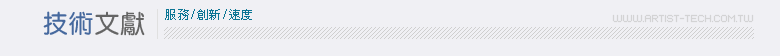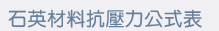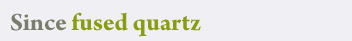Since fused quartz is utilized in applications involving internal pressures, it is sometimes helpful to know the maximum pressure which can be applied to a certain size tube. The following formula will approximate this information at room temperature.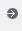CFQ Rupture Formula For Tubing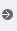CFQ Rupture Formula For Discs And Plates
S = p*r/t
Where:
S = Hoop Stress in Pa
p = Working pressure (Pa)
r = Inside Radius of Tube (mm)
t = Wall Thickness (mm)
This formula is not applicable when
the internal pressure exceeds 7x105 Pa (100 psi).
Calculating pressure differential is also required for many applications of stressed fused quartz discs, plates, and sight glasses.

The formula which follows
can be used for room temperature applications of circular parts with either clamped or unclamped edges.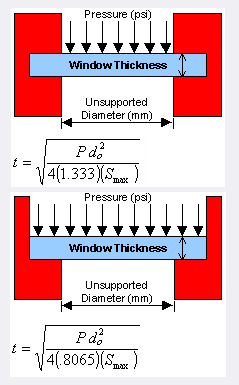Where: p = Pressure differential, PSI do = Unsupported disc diameter, mm (for plates substitute width) Smax = Maximum stress (approx. 7 to 1 safety factor) 1000 PSI t = Disc thickness, mm However the following factors will affect the strength of these parts and must be considered when using the formulae: Surface should be highly polished and free of scratches. Means by which a sample is clamped into a pressure device. The gasketing material used. The thermal gradients expected across the surface and between the surfaces The rate of pressure increase which will be applied. emperature of specimen.